# Axiom of choice

(diff) ← Older revision | Latest revision (diff) | Newer revision → (diff)

One of the axioms in set theory. It states that for any family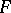of non-empty sets there exists a functionsuch that, for any setfrom, one has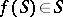(is called a choice function on). For finite familiesthe axiom of choice can be deduced from the other axioms of set theory (e.g. in the system ZF).

The axiom of choice was explicitly formulated by E. Zermelo (1904) and was objected to by many mathematicians. This is explained, first, by its purely existential character which makes it different from the remaining axioms of set theory and, secondly, by some of its implications which are "unacceptable" or even contradict intuitive "common sense" . Thus, the axiom of choice implies: the existence of a Lebesgue non-measurable set of real numbers; the existence of three subdivisions of the sphere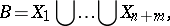such thatis congruent with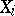,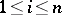, and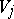is congruent with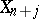,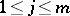. Thus, the sphereis divisible into a finite number of parts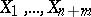which can be moved in space to form two spheres identical to it.

Many postulates equivalent to the axiom of choice were subsequently discovered. Among these are: 1) The well-ordering theorem: On any setthere exists a total order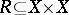such that any non-empty set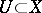contains a least element in the sense of the relation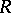; 2) the maximality principle (Zorn's lemma): If any totally ordered subset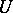of a partially ordered setis bounded from above,contains a maximal element; 3) any non-trivial lattice with a unit element has a maximal ideal; 4) the product of compact topological spaces is compact; and 5) any sethas the same cardinality as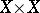.

The axiom of choice does not contradict the other axioms of set theory (e.g. the system ZF) and cannot be logically deduced from them if they are non-contradictory. The axiom of choice is extensively employed in classical mathematics. Thus, it is used in the following theorems. 1) Each subgroup of a free group is free; 2) the algebraic closure of an algebraic field exists and is unique up to an isomorphism; and 3) each vector space has a basis. It is also used in: 4) the equivalence of the two definitions of continuity of a function at a point (the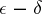-definition and the definition by limits of sequences) and in proving 5) the countable additivity of the Lebesgue measure. The last two theorems follow from the countable axiom of choice (the formulation of the axiom includes the condition of countability of the family). It was proved that the theorems 1) to 5) are not deducible in the system ZF if ZF is non-contradictory.

A model of set theory has been constructed which meets the countable axiom of choice and in which each set of numbers is Lebesgue-measurable. This model was constructed on the assumption that the system ZF does not contradict the axiom of the existence of an inaccessible cardinal number.

How to Cite This Entry:
Axiom of choice. Encyclopedia of Mathematics. URL: http://encyclopediaofmath.org/index.php?title=Axiom_of_choice&oldid=19296
This article was adapted from an original article by V.N. Grishin (originator), which appeared in Encyclopedia of Mathematics - ISBN 1402006098. See original article